List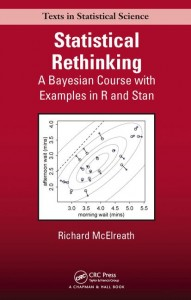Here I work through the practice questions in Chapter 7, “Interactions,” of Statistical Rethinking (McElreath, 2016). I do my best to use only approaches and functions discussed so far in the book, as well as to name objects consistently with how the book does. If you find any typos or mistakes in my answers, or if you have any relevant questions, please feel free to add a comment below.

Here is the chapter summary from page 236:

This chapter introduced interactions, which allow for the association between a predictor and an outcome to depend upon the value of another predictor. Interactions can be difficult to interpret, and so the chapter also introduced triptych plots that help in visualizing the effect of an interaction. No new coding skills were introduced, but the statistical models considered were among the most complicated so far in the book. To go any further, we’re going to need a more capable conditioning engine to fit our models to data. That’s the topic of the next chapter.

# Setup
library(rethinking)
data(tulips)
data(rugged)
data(nettle)
set.seed(7)


## Easy Difficulty

### Practice Question 7E1

For each of the causal relationships below, name a hypothetical third variable that would lead to an interaction effect.

1. Bread dough rises because of yeast.
2. Education leads to higher income.
3. Gasoline makes a car go.
1. Commercial baking yeast is inactive below $$40\,^{\circ}\mathrm{F}$$ and dies above $$130\,^{\circ}\mathrm{F}$$, so temperature would likely interact with yeast to predict amount of bread dough rising.
2. Different fields (e.g., the humanities and engineering) have vastly different distributions of expected income, so field of work (and maybe major if the sample is college-educated) would likely interact with years of education to predict income.
3. Gasoline will only make a working car go, so a variable representing each car’s engine functioning (or repair status) would likely interact with gasoline to predict movement.

### Practice Question 7E2

Which of the following explanations invokes an interaction?

1. Caramelizing onions requires cooking over low heat and making sure the onions do not dry out.
2. A car will go faster when it has more cylinders or when it has a better fuel injector.
3. Most people acquire their political beliefs from their parents, unless they get them instead from their friends.
4. Intelligent animal species tend to be either highly social or have manipulative appendages (hands, tentacles, etc.).
1. This explanation invokes an interaction between heat and dryness in predicting onion caramelization. Specifically, it implies that caramelization will only occur when both heat and dryness are low.
2. As I read it (as containing an “inclusive or”), this explanation invokes main effects of number of cylinders and fuel injector quality on car speed but does not explicitly invoke an interaction. Specifically, it seems to imply that adding cylinders and increasing the quality of the fuel injector are independent routes to increasing car speed.
3. As I read it, this explanation seems to imply that there are two types of people: those who acquire their beliefs from their parents and those who acquire their beliefs from their friends. The implied model seems to predict individuals’ political beliefs using a linear combination of the interactions between type and parents’ beliefs on the one hand and between type and friends’ beliefs on the other hand.
4. As I read it (as containing an “exclusive or”), this explanation seems to invoke an interaction between sociality and the possession of manipulative appendages in predicting a species’ intelligence. Specifically, it seems to imply that intelligent species are high on sociality or have manipulative appendages but are not both high on sociality and in possession of manipulative appendages.

Thus, given my reading, explanations 1, 3, and 4 invoke an interaction.

### Practice Question 7E3

For each of the explanations in 7E2, write a linear model that expresses the stated relationship.

1. $$\mu_i = \beta_H H_i + \beta_D D_i + \beta_{HD} H_i D_i$$
2. $$\mu_i = \beta_C C_i + \beta_Q Q_i$$
3. $$\mu_i = \beta_{TP} T_i P_i + \beta_{TF} T_i F_i$$
4. $$\mu_i = \beta_{S} S_i + \beta_A A_i + \beta_{SA} S_i A_i$$

## Medium Difficulty

### Practice Question 7M1

Recall the tulips example from the chapter. Suppose another set of treatments adjusted the temperature in the greenhouse over two levels: cold and hot. The data in the chapter were collected at the cold temperature. You find none of the plants grown under the hot temperature developed any blooms at all, regardless of the water and shade levels. Can you explain this result in terms of interactions between water, shade, and temperature?

An interaction allows the relationship between a predictor and an outcome to depend upon the value of another predictor. Since the question is stating that the relationships between blossoms and water and between blossoms and shade depend upon the value of temperature, interaction effects can be used here. Since there are three predictor variables now (water, shade, and temperature), we would have a single three-way interaction and three two-way interactions (WST, WS, WT, and ST).

### Practice Question 7M2

Can you invent a regression equation that would make the bloom size zero, whenever the temperature is hot?

The algebraic form of the regression equation would be:
$\mu_i = \alpha + \beta_W W_i + \beta_S S_i + \beta_T T_i + \beta_{WST} W_i S_i T_i + \beta_{WS} W_i S_i + \beta_{WT} W_i T_i + \beta_{ST} S_i T_i$
We can now invent some estimated parameter values that would make the bloom size zero whenever the temperature is hot. To make things simple, let’s assume that all predictors are equal to 1. Doing so makes all the $$W_i$$, $$S_i$$, and $$T_i$$ terms drop out.
$\mu_i|_{W_i=1,S_i=1,T=1} = \alpha + \beta_W + \beta_S + \beta_T + \beta_{WST} + \beta_{WS} + \beta_{WT} + \beta_{ST}$
If we want $$\mu_i$$ to be $$0$$ in this case, then we must counteract the effects of $$\alpha$$, $$\beta_W$$, and $$\beta_S$$ whenever $$T_i=1$$. To do this, we can have $$\beta_T$$ counteract $$\alpha$$, $$\beta_{WT}$$ counteract $$\beta_W$$, $$\beta_{ST}$$ counteract $$\beta_S$$, and $$\beta_{WST}$$ counteract $$\beta_{WS}$$. I will first reorder the equation above to put the $$T$$ term directly after whatever it counteracts.
$\mu_i|_{W_i=1,S_i=1,T=1} = (\alpha + \beta_T) + (\beta_W + \beta_{WT}) + (\beta_S + \beta_{ST}) + (\beta_{WS} + \beta_{WST})$

Now we can substitute in the counteracting terms. For example, we set $$\beta_T$$ equal to $$-\alpha$$ and we set $$\beta_{WT}$$ equal to $$-\beta_W$$.
$\mu_i|_{W_i=1,S_i=1,T=1} = (\alpha – \alpha) + (\beta_W – \beta_W) + (\beta_S – \beta_S) + (\beta_{WS} – \beta_{WS})$

Hopefully it is clear why, in this case, $$\mu_i=0$$. Because all the terms involving $$T$$ will drop out when $$T_i=0$$, the full regression equation will perform the same as it did before temperature was introduced.
$\mu_i = \alpha + \beta_W W_i + \beta_S S_i -\alpha T_i – \beta_{WS} W_i S_i T_i + \beta_{WS} W_i S_i – \beta_{W} W_i T_i – \beta_{S} S_i T_i$
If we trust the estimates provided on page 232, we can even put the exact numbers in.
$\mu_i = 129.01 + (74.96) W_i + (-41.14) S_i + (-129.01) T_i + (51.87) W_i S_i T_i + (-51.87) W_i S_i + (-74.96) W_i T_i + (41.14) S_i T_i$

### Practice Question 7M3

In parts of North America, ravens depend upon wolves for their food. This is because ravens are carnivorous but cannot usually kill or open carcasses of prey. Wolves however can and do kill and tear open animals, and they tolerate ravens co-feeding at their kills. This species relationship is generally described as a “species interaction.” Can you invent a hypothetical set of data on raven population size in which this relationship would manifest as a statistical interaction? Do you think the biological interaction could be linear? Why or why not?

We need to invent some data in which prey population and wolf population interact in predicting raven population. The linear model here would be the following:
$\mu_i = \alpha + \beta_P P_i + \beta_W W_i + \beta_{PW} P_i W_i$

In order for invented data to manifest this relationship as a statistical interaction, observations (e.g., areas) would need to have generally high raven populations only when there were also high prey and wolf populations (as neither alone would be enough to support a high raven population). Let’s simulate such data and, for simplicity, assume standardized variables. There are several approaches we could have used here, but the most straightforward approach that the book has already presented is an expansion of what is presented on page 141.

Let’s start with prey and assume their populations are normally distributed across areas. Then let’s can assume that wolves are attracted to areas with high prey populations but that they then kill or drive away many prey. Therefore there is a positive but not a perfect correlation between prey and wolf populations. We can further assume that ravens are attracted to areas in which prey or wolf populations are high and especially areas in which both are high. Since there are probably predators other than wolves that kill prey, we will make the main effect for prey higher than the main effect for wolf.

N <- 300 # simulation size
rPW <- 0.6 # correlation between prey and wolf
bP <- 0.3 # regression coefficient for prey
bW <- 0.1 # regression coefficient for wolf
bPW <- 0.5 # regression coefficient for prey-by-wolf interaction
# Simulate data
prey <- rnorm(
n = N,
mean = 0,
sd = 1
)
wolf <- rnorm(
n = N,
mean = rPW * prey,
sd = sqrt(1 - rPW^2)
)
raven <- rnorm(
n = N,
mean = bP*prey + bW*wolf + bPW*prey*wolf,
sd = 1
)
d <- data.frame(raven, prey, wolf)
str(d)

## 'data.frame':    300 obs. of  3 variables:
##  $raven: num 1.6876 0.2429 -0.4894 -0.0254 0.2049 ... ##$ prey : num  2.287 -1.197 -0.694 -0.412 -0.971 ...
d$water.c <- d$water - mean(d$water) # Dummy variables d$bedb <- d$bed == "b" d$bedc <- d$bed == "c" # Index variable d$bedx <- coerce_index(d$bed)  First let’s do it with dummy variables. m_dummy <- map( alist( blooms ~ dnorm(mu, sigma), mu <- a + bW*water.c + bS*shade.c + bWS*water.c*shade.c + bBb*bedb + bBc*bedc, a ~ dnorm(130, 100), bW ~ dnorm(0, 100), bS ~ dnorm(0, 100), bWS ~ dnorm(0, 100), bBb ~ dnorm(0, 100), bBc ~ dnorm(0, 100), sigma ~ dunif(0, 100) ), data = d, start = list(a = mean(d$blooms), bW = 0, bS = 0, bWS = 0, bBb = 0, bBc = 0, sigma = sd(d$blooms)) ) precis(m_dummy)  ## Mean StdDev 5.5% 94.5% ## a 99.36 12.76 78.97 119.75 ## bW 75.12 9.20 60.42 89.83 ## bS -41.23 9.20 -55.93 -26.53 ## bWS -52.15 11.24 -70.12 -34.18 ## bBb 42.41 18.04 13.58 71.24 ## bBc 47.03 18.04 18.20 75.86 ## sigma 39.19 5.34 30.66 47.72  Now let’s repeat using an index variable. It is important the remember the depth=2 argument for the precis() function or else we will get an error. m_index <- map( alist( blooms ~ dnorm(mu, sigma), mu <- a[bedx] + bW*water.c + bS*shade.c + bWS*water.c*shade.c, a[bedx] ~ dnorm(130, 100), bW ~ dnorm(0, 100), bS ~ dnorm(0, 100), bWS ~ dnorm(0, 100), sigma ~ dunif(0, 200) ), data = d ) precis(m_index, depth = 2)  ## Mean StdDev 5.5% 94.5% ## a 97.67 12.95 76.97 118.37 ## a 142.37 12.95 121.67 163.07 ## a 146.99 12.95 126.29 167.69 ## bW 75.16 9.20 60.46 89.86 ## bS -41.25 9.20 -55.95 -26.55 ## bWS -52.18 11.24 -70.15 -34.22 ## sigma 39.18 5.33 30.66 47.71  The results are very similar, with the main differences being the form in which the bed-specific intercepts are presented. In the dummy variable approach, a is the intercept for bed “a”, and the get the intercepts for beds “b” and “c” you have to add the bBb and bBc coefficients to a. In the index variable approach, the computation is done for us and a is the intercept for bed “a”, a is the intercept for bed “b”, and a is the intercept for bed “c”. coeftab(m_dummy, m_index)  ## m_dummy m_index ## a 99.36 NA ## bW 75.12 75.16 ## bS -41.23 -41.25 ## bWS -52.15 -52.18 ## bBb 42.41 NA ## bBc 47.03 NA ## sigma 39.19 39.18 ## a NA 97.67 ## a NA 142.37 ## a NA 146.99 ## nobs 27 27  ### Practice Question 7H2 Use WAIC to compare the model from 7H1 to a model that omits bed. What do you infer from this comparison? Can you reconcile the WAIC results with the posterior distribution of the bed coefficients? We can just copy down the example from before and remove the bed information: m_omit <- map( alist( blooms ~ dnorm(mu, sigma), mu <- a + bW*water.c + bS*shade.c + bWS*water.c*shade.c, a ~ dnorm(130, 100), bW ~ dnorm(0, 100), bS ~ dnorm(0, 100), bWS ~ dnorm(0, 100), sigma ~ dunif(0, 100) ), data = d, start = list(a = mean(d$blooms), bW = 0, bS = 0, bWS = 0, sigma = sd(d$blooms)) ) precis(m_omit)  ## Mean StdDev 5.5% 94.5% ## a 129.01 8.67 115.15 142.87 ## bW 74.96 10.60 58.02 91.90 ## bS -41.14 10.60 -58.08 -24.20 ## bWS -51.87 12.95 -72.57 -31.18 ## sigma 45.22 6.15 35.39 55.06  Now, let’s compare them using WAIC: compare(m_dummy, m_omit)  ## WAIC pWAIC dWAIC weight SE dSE ## m_dummy 294.6 9.7 0.0 0.67 10.03 NA ## m_omit 296.0 6.6 1.4 0.33 10.57 7.56  The model including the bed dummy variables had a better WAIC than the model that omitted the bed variable, and most of the Akaike weight. I infer from this, as well as from the bed intercepts, that there was a lot of variability in bloom size between beds. Bed “a” had particularly fewer blooms than the other beds. This is visible in the estimates table, but it is even clearer in a posterior distribution plot (pages 221 and 222). post <- extract.samples(m_dummy) post.a <- post$a
post.b <- post$a + post$bBb
post.c <- post$a + post$bBc
dens(post.a, col = "red", xlim = c(50, 200), ylim = c(0, 0.035))
dens(post.b, col = "blue", add = TRUE)
dens(post.c, col = "black", add = TRUE)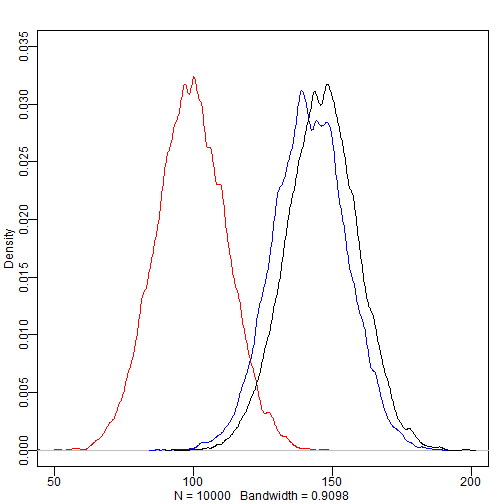### Practice Question 7H3

Consider again the data(rugged) data on economic development and terrain ruggedness, examined in this chapter. One of the African countries in that sample, Seychelles, is far outside the cloud of other nations, being a rare country with both relatively high GDP and high ruggedness. Seychelles is also unusual, in that it is a group of islands far from the coast of mainland Africa, and its main economic activity in tourism.

One might suspect that this one nation is exerting a strong influence on the conclusions. In this problem, I want you to drop Seychelles from the data and re-evaluate the hypothesis that the relationship of African economies with ruggedness is different from that on other continents.

(a) Begin by using map() to fit just the interaction model:
\begin{aligned} y_i &\sim \mathrm{Normal}(\mu_i, \sigma) \\ \mu_i &= \alpha + \beta_A A_i + \beta_R R_i + \beta_{AR} A_i R_i \end{aligned}
where $$y$$ is log GDP per capita in the year 2000 (log of rgdppc_2000); $$A$$ is cont_africa, the dummy variable for being an African nation; and $$R$$ is the variable rugged. Choose your own priors. Compare the inference from this model fit to the data without Seychelles to the same model fit to the full data. Does it still seem like the effect of ruggedness depends upon continent? How much has the expected relationship changed?

(b) Now plot the predictions of the interaction model, with and without Seychelles. Does it still seem like the effect of ruggedness depends upon continent? How much has the expected relationship changed?

(c) Finally, conduct a model comparison analysis, using WAIC. Fit three models to the data without Seychelles:
\begin{aligned}
\mathrm{Model 1:}\; y_i &\sim \mathrm{Normal}(\mu_i, \sigma) \\
\mu_i &= \alpha + \beta_R R_i \\
\mathrm{Model 2:}\; y_i &\sim \mathrm{Normal}(\mu_i, \sigma) \\
\mu_i &= \alpha + \beta_A A_i + \beta_R R_i \\
\mathrm{Model 3:}\; y_i &\sim \mathrm{Normal}(\mu_i, \sigma) \\
\mu_i &= \alpha + \beta_A A_i + \beta_R R_i + \beta_{AR} A_i R_i \\
\end{aligned}
Use whatever priors you think are sensible. Plot the model-averaged predictions of this model set. Do your inferences differ from those in (b)? Why or why not?

For part (a), let’s create dataframes with and without Seychelles and then fit the specified interaction model to both (page 217).

d <- rugged[complete.cases(rugged$rgdppc_2000), ] d$log_gdp <- log(d$rgdppc_2000) d2 <- d[d$country != "Seychelles", ]
m_with <- map(
alist(
log_gdp ~ dnorm(mu, sigma),
mu <- a + bA*cont_africa + bR*rugged + bAR*cont_africa*rugged,
a ~ dnorm(8, 100),
bA ~ dnorm(0, 1),
bR ~ dnorm(0, 1),
bAR ~ dnorm(0, 1),
sigma ~ dunif(0, 10)
),
data = d
)
m_without <- map(
alist(
log_gdp ~ dnorm(mu, sigma),
mu <- a + bA*cont_africa + bR*rugged + bAR*cont_africa*rugged,
a ~ dnorm(8, 100),
bA ~ dnorm(0, 1),
bR ~ dnorm(0, 1),
bAR ~ dnorm(0, 1),
sigma ~ dunif(0, 10)
),
data = d2
)
coeftab(m_with, m_without)

##       m_with  m_without
## a        9.18    9.19
## bA      -1.85   -1.78
## bR      -0.18   -0.19
## bAR      0.35    0.25
## sigma    0.93    0.93
## nobs      170     169


Comparing this table to the results on pages 220 and 221, two changes are notable. The first is that bA dropped a bit and the second is that bAR dropped from 0.35 to 0.25. The latter is a rather large difference, especially considering the size of its SE. From only looking at these estimates, I am less confident that the effect of ruggedness depends upon continent. Or perhaps it still does, but the dependency is smaller than expected.

For part (b), we need to plot the predictions of both models (page 219).

par(mfrow = c(2, 2))
# Predictions for model with Seychelles
rugged.seq <- seq(from = -1, to = 8, by = 0.25)
mu.Africa <- link(m_with, data = data.frame(cont_africa = 1, rugged = rugged.seq), refresh = 0)
mu.Africa.mean <- apply(mu.Africa, 2, mean)
mu.Africa.PI <- apply(mu.Africa, 2, PI, prob = 0.97)
mu.NotAfrica <- link(m_with, data = data.frame(cont_africa = 0, rugged = rugged.seq), refresh = 0)
mu.NotAfrica.mean <- apply(mu.NotAfrica, 2, mean)
mu.NotAfrica.PI <- apply(mu.NotAfrica, 2, PI, prob = 0.97)
d.A1 <- d[d$cont_africa == 1, ] plot(log_gdp ~ rugged, data = d.A1, col = rangi2) mtext("African nations, with Seychelles", 3) lines(rugged.seq, mu.Africa.mean, col = rangi2) shade(mu.Africa.PI, rugged.seq, col = col.alpha(rangi2, 0.3)) d.A0 <- d[d$cont_africa == 0, ]
plot(log_gdp ~ rugged, data = d.A0)
mtext("Non-African nations, with Seychelles", 3)
lines(rugged.seq, mu.NotAfrica.mean)
# Predictions for model without Seychelles
rugged.seq <- seq(from = -1, to = 8, by = 0.25)
mu.Africa <- link(m_without, data = data.frame(cont_africa = 1, rugged = rugged.seq), refresh = 0)
mu.Africa.mean <- apply(mu.Africa, 2, mean)
mu.Africa.PI <- apply(mu.Africa, 2, PI, prob = 0.97)
mu.NotAfrica <- link(m_without, data = data.frame(cont_africa = 0, rugged = rugged.seq), refresh = 0)
mu.NotAfrica.mean <- apply(mu.NotAfrica, 2, mean)
mu.NotAfrica.PI <- apply(mu.NotAfrica, 2, PI, prob = 0.97)
d.A1 <- d2[d2$cont_africa == 1, ] plot(log_gdp ~ rugged, data = d.A1, col = rangi2) mtext("African nations, without Seychelles", 3) lines(rugged.seq, mu.Africa.mean, col = rangi2) shade(mu.Africa.PI, rugged.seq, col = col.alpha(rangi2, 0.3)) d.A0 <- d2[d2$cont_africa == 0, ]
plot(log_gdp ~ rugged, data = d.A0)
mtext("Non-African nations, without Seychelles", 3)
lines(rugged.seq, mu.NotAfrica.mean)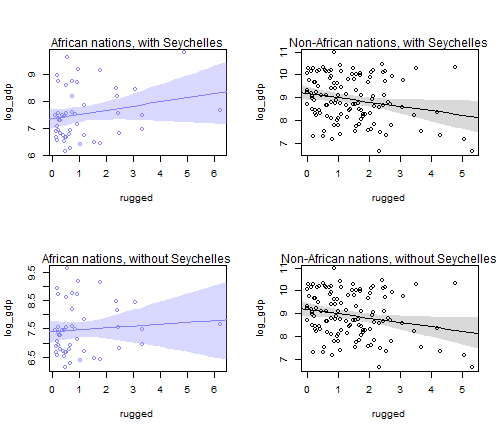Without Seychelles, the slope among African nations is a bit shallower and the uncertainty at the higher values of terrain ruggedness is larger. The plots make it seem that the interaction is rather subtle/weak with Seychelles and especially without it.

Finally, for part (c), we need to fit three increasingly complex models and compare them using WAIC, both with and without Seychelles. We can reuse the d and d2 dataframes we created for part (a).

m_with1 <- map(
alist(
log_gdp ~ dnorm(mu, sigma),
mu <- a + bR*rugged,
a ~ dnorm(8, 100),
bR ~ dnorm(0, 1),
sigma ~ dunif(0, 10)
),
data = d
)
m_with2 <- map(
alist(
log_gdp ~ dnorm(mu, sigma),
mu <- a + bA*cont_africa + bR*rugged,
a ~ dnorm(8, 100),
bA ~ dnorm(0, 1),
bR ~ dnorm(0, 1),
sigma ~ dunif(0, 10)
),
data = d
)
m_with3 <- map(
alist(
log_gdp ~ dnorm(mu, sigma),
mu <- a + bA*cont_africa + bR*rugged + bAR*cont_africa*rugged,
a ~ dnorm(8, 100),
bA ~ dnorm(0, 1),
bR ~ dnorm(0, 1),
bAR ~ dnorm(0, 1),
sigma ~ dunif(0, 10)
),
data = d
)
compare(m_with1, m_with2, m_with3)

##          WAIC pWAIC dWAIC weight    SE   dSE
## m_with3 469.7   5.3   0.0   0.97 15.17    NA
## m_with2 476.4   4.5   6.7   0.03 15.30  6.18
## m_with1 539.4   2.6  69.7   0.00 13.31 15.27

m_without1 <- map(
alist(
log_gdp ~ dnorm(mu, sigma),
mu <- a + bR*rugged,
a ~ dnorm(8, 100),
bR ~ dnorm(0, 1),
sigma ~ dunif(0, 10)
),
data = d2
)
m_without2 <- map(
alist(
log_gdp ~ dnorm(mu, sigma),
mu <- a + bA*cont_africa + bR*rugged,
a ~ dnorm(8, 100),
bA ~ dnorm(0, 1),
bR ~ dnorm(0, 1),
sigma ~ dunif(0, 10)
),
data = d2
)
m_without3 <- map(
alist(
log_gdp ~ dnorm(mu, sigma),
mu <- a + bA*cont_africa + bR*rugged + bAR*cont_africa*rugged,
a ~ dnorm(8, 100),
bA ~ dnorm(0, 1),
bR ~ dnorm(0, 1),
bAR ~ dnorm(0, 1),
sigma ~ dunif(0, 10)
),
data = d2
)
compare(m_without1, m_without2, m_without3)

##             WAIC pWAIC dWAIC weight    SE   dSE
## m_without3 463.1   4.4   0.0   0.83 14.90    NA
## m_without2 466.3   4.0   3.2   0.17 14.20  3.33
## m_without1 536.1   2.7  73.0   0.00 13.45 15.11


The models with Seychelles included have more of the Akaike weight assigned to the model with the interaction term (0.96) than do the models without Seychelles (0.79). This suggests that Seychelles was influential in making the interaction term seem important.

Finally, let’s plot the model-averaged predictions for these two sets of models (pages 203 and 204).

par(mfrow = c(2, 2))
# Plots from ensemble on data with Seychelles
rugged.seq <- seq(from = -1, to = 8, by = 0.25)
dA.predict <- list(
log_gdp = rep(0, 37),
rugged = rugged.seq,
cont_africa = rep(1, 37)
)
dN.predict <- list(
log_gdp = rep(0, 37),
rugged = rugged.seq,
cont_africa = rep(0, 37)
)
withA_ensemble <- ensemble(m_with1, m_with2, m_with3, data = dA.predict, refresh = 0)
withN_ensemble <- ensemble(m_with1, m_with2, m_with3, data = dN.predict, refresh = 0)
muA <- apply(withA_ensemble$link, 2, mean) muA.PI <- apply(withA_ensemble$link, 2, PI, prob = 0.97)
muN <- apply(withN_ensemble$link, 2, mean) muN.PI <- apply(withN_ensemble$link, 2, PI, prob = 0.97)
dA <- d[d$cont_africa == 1, ] plot(log_gdp ~ rugged, data = dA, col = rangi2) lines(rugged.seq, muA, col = rangi2) shade(muA.PI, rugged.seq, col = col.alpha(rangi2, 0.3)) mtext("African nations, with Seychelles", 3) dN <- d[d$cont_africa == 0, ]
plot(log_gdp ~ rugged, data = dN, col = rangi2)
lines(rugged.seq, muN)
mtext("Non-African nations, with Seychelles", 3)
# Plots from ensemble on data without Seychelles
dA.predict <- list(
log_gdp = rep(0, 37),
rugged = rugged.seq,
cont_africa = rep(1, 37)
)
dN.predict <- list(
log_gdp = rep(0, 37),
rugged = rugged.seq,
cont_africa = rep(0, 37)
)
withoutA_ensemble <- ensemble(m_without1, m_without2, m_without3, data = dA.predict, refresh = 0)
withoutN_ensemble <- ensemble(m_without1, m_without2, m_without3, data = dN.predict, refresh = 0)
muA <- apply(withoutA_ensemble$link, 2, mean) muA.PI <- apply(withoutA_ensemble$link, 2, PI, prob = 0.97)
muN <- apply(withoutN_ensemble$link, 2, mean) muN.PI <- apply(withoutN_ensemble$link, 2, PI, prob = 0.97)
dA <- d2[d2$cont_africa == 1, ] plot(log_gdp ~ rugged, data = dA, col = rangi2) lines(rugged.seq, muA, col = rangi2) shade(muA.PI, rugged.seq, col = col.alpha(rangi2, 0.3)) mtext("African nations, without Seychelles", 3) dN <- d2[d2$cont_africa == 0, ]
plot(log_gdp ~ rugged, data = dN, col = rangi2)
lines(rugged.seq, muN)
mtext("Non-African nations, without Seychelles", 3)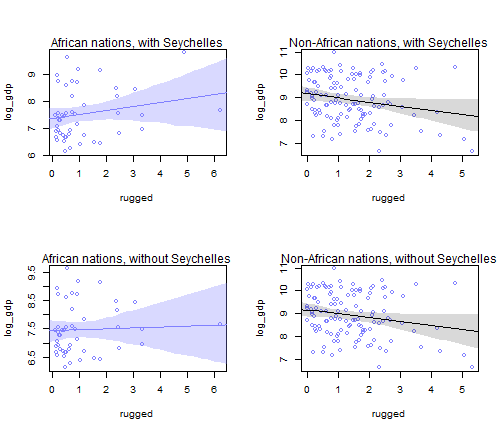Because Model 2 (which has no interaction term) is afforded more Akaike weight when Seychelles is excluded, the posterior predictions of the ensemble are even more consistent with the lack of an interaction effect. The slope looks nearly flat and the interval estimates are so wide that they easily included the slope found for the non-African countries. This further undermines my confidence that the relation between GDP and terrain ruggedness depends on continent.

### Practice Question 7H4

The values in data(nettle) are data on language diversity in 74 nations. The meaning of each column is given below.

(1) country: Name of the country

(2) num.lang: Number of recognized languages spoken

(3) area: Area in square kilometers

(4) k.pop: Population, in thousands

(5) num.stations: Number of weather stations that provided data for the next two columns

(6) mean.growing.season: Average length of growing season, in months

(7) sd.growing.season: Standard deviation of length of growing season, in months

Use these data to evaluate the hypothesis that language diversity is partly a product of food security. The notion is that, in productive ecologies, people don’t need large social networks to buffer them against risk of food shortfalls. This means ethnic groups can be smaller and more self-sufficient, leading to more languages per capita. In contrast, in a poor ecology, there is more subsistence risk, and so human societies have adapted by building larger networks of mutual obligation to provide food insurance. This in turn creates social forces that help prevent languages from diversifying.

Specifically, you will try to model the number of languages per capita as the outcome variable.

d$lang.per.cap <- d$num.lang / d$k.pop Use the logarithm of this new variable as your regression outcome. (A count model would be better here, but you’ll learn those later, in Chapter 10.) This problem is open ended, allowing you to decide how you address the hypotheses and the uncertain advice the modeling provides. If you think you need to use WAIC anyplace, please do. If you think you need certain priors, argue for them. If you think you need to plot predictions in a certain way, please do. Just try to honestly evaluate the main effects of both mean.growing.season and sd.growing.season, as well as their two-way interaction, as outlined in parts (a), (b), and © below. If you are not sure which approach to use, try several. (a) Evaluate the hypothesis that language diversity, as measured by log(lang.per.cap), is positively associated with the average length of the growing season mean.growing.season. Consider log(area) in your regression(s) as a covariate (not an interaction). Interpret your results. (b) Now evaluate the hypothesis that language diversity is negatively associated with the standard deviation of length of growing seasons, sd.growing.season. This hypothesis follows from uncertainty in harvest favoring social insurance through larger social networks and therefore fewer languages. Again, consider log(area) as a covariate (not an interaction). Interpret your results. (c) Finally, evaluate the hypothesis that mean.growing.season and sd.growing.seasons interact to synergistically reduce language diversity. The idea is that, in nations with longer average growing seasons, high variance makes storage and redistribution even more important than it would be otherwise. That way, people can cooperate to preserve and protect windfalls to be used during the draughts. These forces in turn may lead to greater social integration and fewer languages. For part (a), I’ll begin by regressing language diversity on the average length of the growing season. d <- nettle d$lang.per.cap <- d$num.lang / d$k.pop
d$log_lpc <- log(d$lang.per.cap)
d$log_area <- log(d$area)
d$log_area.c <- d$log_area - mean(d$log_area) d$mgs.c <- d$mean.growing.season - mean(d$mean.growing.season)
d$sgs.c <- d$sd.growing.season - mean(d$sd.growing.season) m_a1 <- map( alist( log_lpc ~ dnorm(mu, sigma), mu <- a + bM*mgs.c, a ~ dnorm(-5, 5), bM ~ dnorm(0, 5), sigma ~ dunif(0, 10) ), data = d ) precis(m_a1)  ## Mean StdDev 5.5% 94.5% ## a -5.46 0.16 -5.72 -5.19 ## bM 0.17 0.05 0.09 0.26 ## sigma 1.41 0.12 1.22 1.59  I’ll also plot the posterior predictions since that was helpful last time. mgsc.seq <- seq(from = -10, to = 10, by = 0.25) mu <- link(m_a1, data = data.frame(mgs.c = mgsc.seq), refresh = 0) mu.mean <- apply(mu, 2, mean) mu.PI <- apply(mu, 2, PI, prob = 0.97) plot(log_lpc ~ mgs.c, data = d, col = rangi2) lines(mgsc.seq, mu.mean) shade(mu.PI, mgsc.seq)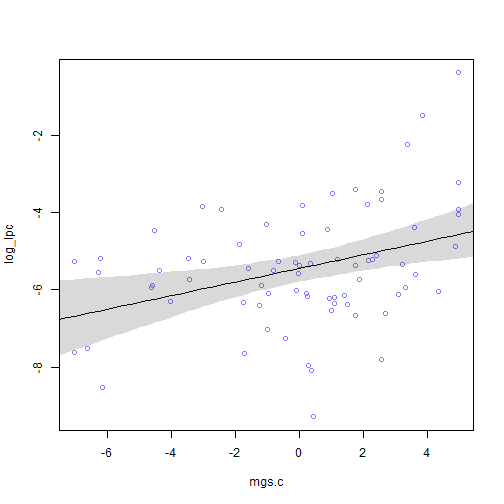This regression model seems to support the hypothesis that language diversity is positively associated with the average length of growing seasons. However, the question asked us to also consider a model with area included. So let’s do the same: m_a2 <- map( alist( log_lpc ~ dnorm(mu, sigma), mu <- a + bM*mgs.c + bA*log_area.c, a ~ dnorm(-5, 5), bM ~ dnorm(0, 5), bA ~ dnorm(0, 5), sigma ~ dunif(0, 10) ), data = d ) precis(m_a2)  ## Mean StdDev 5.5% 94.5% ## a -5.46 0.16 -5.71 -5.20 ## bM 0.14 0.06 0.05 0.23 ## bA -0.20 0.14 -0.42 0.02 ## sigma 1.39 0.11 1.21 1.57  mgsc.seq <- seq(from = -10, to = 10, length.out = 50) mu <- link(m_a2, data = data.frame(mgs.c = mgsc.seq, log_area.c = 0), refresh = 0) mu.mean <- apply(mu, 2, mean) mu.PI <- apply(mu, 2, PI, prob = 0.97) plot(log_lpc ~ mgs.c, data = d, col = rangi2) lines(mgsc.seq, mu.mean) shade(mu.PI, mgsc.seq)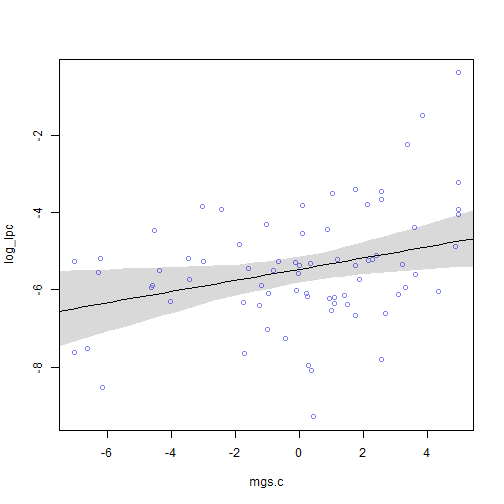coeftab(m_a1, m_a2)  ## m_a1 m_a2 ## a -5.46 -5.46 ## bM 0.17 0.14 ## sigma 1.41 1.39 ## bA NA -0.2 ## nobs 74 74  compare(m_a1, m_a2)  ## WAIC pWAIC dWAIC weight SE dSE ## m_a1 267.7 3.6 0.0 0.54 15.08 NA ## m_a2 268.0 4.9 0.3 0.46 15.73 3.73  In this model, which includes area, the mean length of growing season was slightly reduced (from 0.17 to 0.14) but not by too much. And the Akaike weights slightly favor the first model without area. To be conservative, I think model-averaging makes sense here. d.predict <- list( log_lpc = rep(0, 50), mgs.c = mgsc.seq, log_area.c = rep(0, 50) ) ma_ensemble <- ensemble(m_a1, m_a2, data = d.predict, refresh = 0) mu <- apply(ma_ensemble$link, 2, mean)
mu.PI <- apply(ma_ensemble$link, 2, PI, prob = 0.97) plot(log_lpc ~ mgs.c, data = d, col = rangi2) lines(mgsc.seq, mu) shade(mu.PI, mgsc.seq)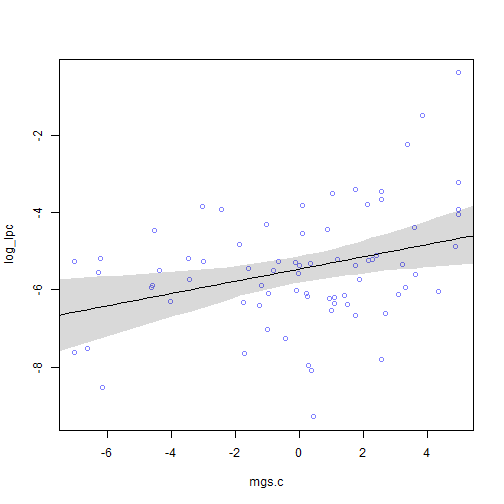Looking at this graph, it seems like a positive association is possible, although a slope of zero is close to the interval estimate if not within it. For part (b), we can repeat the same steps substituting in the SD of the length of growing seasons for the mean. m_b1 <- map( alist( log_lpc ~ dnorm(mu, sigma), mu <- a + bS*sgs.c, a ~ dnorm(-5, 5), bS ~ dnorm(0, 5), sigma ~ dunif(0, 10) ), data = d ) precis(m_b1)  ## Mean StdDev 5.5% 94.5% ## a -5.46 0.17 -5.73 -5.18 ## bS -0.36 0.16 -0.62 -0.10 ## sigma 1.46 0.12 1.27 1.65  sgsc.seq <- seq(from = -5, to = 10, length.out = 50) mu <- link(m_b1, data = data.frame(sgs.c = sgsc.seq), refresh = 0) mu.mean <- apply(mu, 2, mean) mu.PI <- apply(mu, 2, PI, prob = 0.97) plot(log_lpc ~ sgs.c, data = d, col = rangi2) lines(sgsc.seq, mu.mean) shade(mu.PI, sgsc.seq)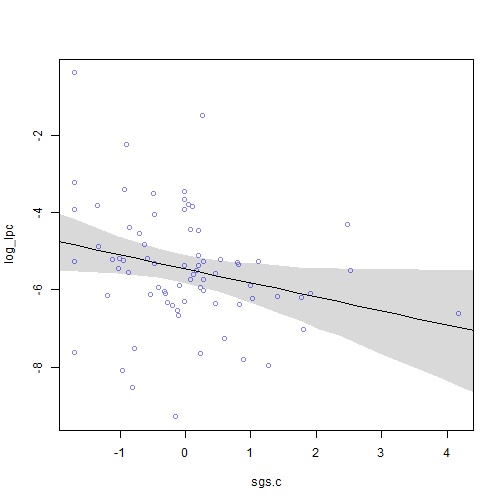And again, controlling for area: m_b2 <- map( alist( log_lpc ~ dnorm(mu, sigma), mu <- a + bS*sgs.c + bA*log_area.c, a ~ dnorm(-5, 5), bS ~ dnorm(0, 5), bA ~ dnorm(0, 5), sigma ~ dunif(0, 10) ), data = d ) precis(m_b2)  ## Mean StdDev 5.5% 94.5% ## a -5.46 0.17 -5.72 -5.19 ## bS -0.21 0.19 -0.51 0.09 ## bA -0.24 0.16 -0.49 0.01 ## sigma 1.44 0.12 1.25 1.63  sgsc.seq <- seq(from = -5, to = 10, length.out = 50) mu <- link(m_b2, data = data.frame(sgs.c = sgsc.seq, log_area.c = 0), refresh = 0) mu.mean <- apply(mu, 2, mean) mu.PI <- apply(mu, 2, PI, prob = 0.97) plot(log_lpc ~ sgs.c, data = d, col = rangi2) lines(sgsc.seq, mu.mean) shade(mu.PI, sgsc.seq)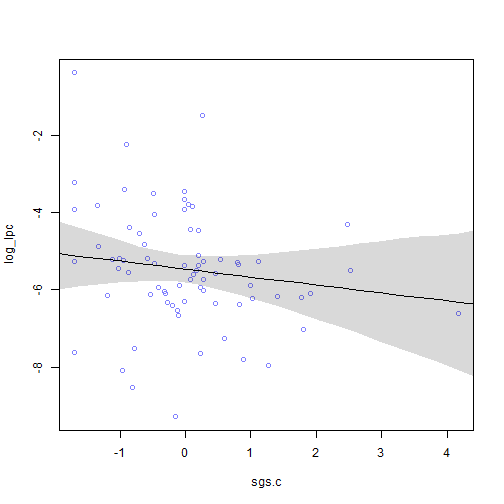coeftab(m_b1, m_b2)  ## m_b1 m_b2 ## a -5.46 -5.46 ## bS -0.36 -0.21 ## sigma 1.46 1.44 ## bA NA -0.24 ## nobs 74 74  compare(m_b1, m_b2)  ## WAIC pWAIC dWAIC weight SE dSE ## m_b1 274.1 4.2 0.0 0.52 17.27 NA ## m_b2 274.2 5.6 0.2 0.48 17.21 3.97  The story is similar for the SD models. There appears to be a negative relationship between language diversity and SD of length of growing seasons and the Akaike weights slightly favor the model without area. However, when controlling for area, the SD slope is reduced a lot in magnitude (-0.36 to 0.21). Thus, I am less confident in this finding. Given the near equal weighting of models, model-averaging seems warranted. d.predict <- list( log_lpc = rep(0, 50), sgs.c = sgsc.seq, log_area.c = rep(0, 50) ) mb_ensemble <- ensemble(m_b1, m_b2, data = d.predict, refresh = 0)  mu <- apply(mb_ensemble$link, 2, mean)
mu.PI <- apply(mb_ensemble$link, 2, PI, prob = 0.97) plot(log_lpc ~ sgs.c, data = d, col = rangi2) lines(sgsc.seq, mu) shade(mu.PI, sgsc.seq)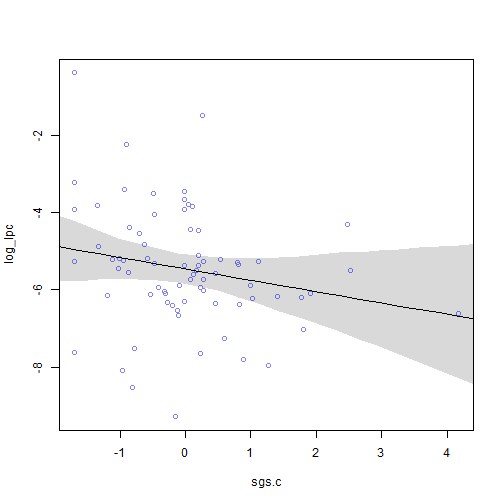This again looks like a negative relationship is possible, but a zero slope is definitely in the interval estimate. So I’m not convinced that there is a negative relationship between language diversity and the SD of the length of growing seasons. Finally, for part ©, we need to examine the interaction of the mean and SD of the length of growing seasons. m_c1 <- map( alist( log_lpc ~ dnorm(mu, sigma), mu <- a + bM*mgs.c + bS*sgs.c + bMS*mgs.c*sgs.c, a ~ dnorm(-5, 5), bM ~ dnorm(0, 5), bS ~ dnorm(0, 5), bMS ~ dnorm(0, 5), sigma ~ dunif(0, 10) ), data = d ) precis(m_c1)  ## Mean StdDev 5.5% 94.5% ## a -5.45 0.15 -5.69 -5.21 ## bM 0.11 0.06 0.03 0.20 ## bS -0.34 0.14 -0.57 -0.11 ## bMS -0.11 0.05 -0.18 -0.03 ## sigma 1.31 0.11 1.14 1.48  The estimates table looks promising for an interaction, but we should really plot the posterior predictions using a triptych plot since continuous variable interactions are so difficult to interpret (page 233). The trick here (which wasn’t included in the book to my knowledge) is to discretize one of the variables (e.g., using cut()). par(mfrow = c(2, 3)) # Discretize variables into groups d$mgsc.group <- cut(
d$mgs.c, breaks = quantile(d$mgs.c, probs = c(0, 1/3, 2/3, 1)),
include.lowest = TRUE,
dig.lab = 2
)
d$sgsc.group <- cut( d$sgs.c,
breaks = quantile(d$sgs.c, probs = c(0, 1/3, 2/3, 1)), include.lowest = TRUE, dig.lab = 2 ) # Plot first row as mean against SD mgsc.seq <- seq(from = -10, to = 10, length.out = 50) for (group in levels(d$sgsc.group)) {
dt <- d[d$sgsc.group == group, ] plot(log_lpc ~ mgs.c, data = dt, col = rangi2, xlim = c(-8, 6), ylim = c(-10, 0), main = paste("SD GS = ", group), xlab = "Mean GS") mu <- link(m_c1, data = data.frame(mgs.c = mgsc.seq, sgs.c = mean(dt$sgs.c)),
refresh = 0)
mu.mean <- apply(mu, 2, mean)
mu.PI <- apply(mu, 2, PI, prob = 0.97)
lines(mgsc.seq, mu.mean)
lines(mgsc.seq, mu.PI[1, ], lty = 2)
lines(mgsc.seq, mu.PI[2, ], lty = 2)
}
# Plot second row as SD against mean
sgsc.seq <- seq(from = -5, to = 10, length.out = 50)
for (group in levels(d$mgsc.group)) { dt <- d[d$mgsc.group == group, ]
plot(log_lpc ~ sgs.c, data = dt, col = rangi2, xlim = c(-2, 5), ylim = c(-10, 0),
main = paste("Mean GS = ", group), xlab = "SD GS")
mu <- link(m_c1, data = data.frame(sgs.c = sgsc.seq, mgs.c = mean(dt\$mgs.c)),
refresh = 0)
mu.mean <- apply(mu, 2, mean)
mu.PI <- apply(mu, 2, PI, prob = 0.97)
lines(sgsc.seq, mu.mean)
lines(sgsc.seq, mu.PI[1, ], lty = 2)
lines(sgsc.seq, mu.PI[2, ], lty = 2)
}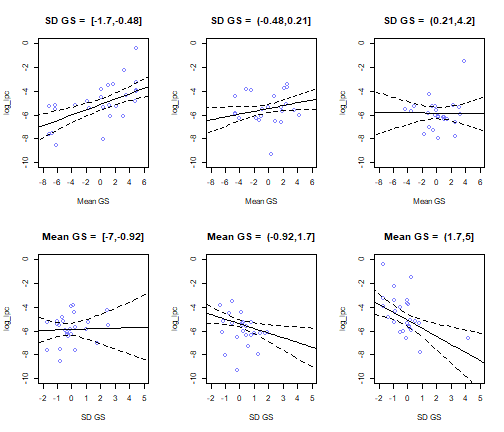These plots show that the association between mean length of growing season and language diversity is positive when SD length of growing season is low, but is basically zero when SD length of growing season is high. Similarly, the association between SD length of growing season and language diversity is basically zero when mean length of growing season is low, but is negative when mean length of growing season is high. This is consistent with the hypothesis presented in the question.

However, we should probably repeat this while controlling for area.

m_c2 <- map(
alist(
log_lpc ~ dnorm(mu, sigma),
mu <- a + bM*mgs.c + bS*sgs.c + bMS*mgs.c*sgs.c + bA*log_area.c,
a ~ dnorm(-5, 5),
bM ~ dnorm(0, 5),
bS ~ dnorm(0, 5),
bMS ~ dnorm(0, 5),
bA ~ dnorm(0, 5),
sigma ~ dunif(0, 10)
),
data = d
)
precis(m_c2)

##        Mean StdDev  5.5% 94.5%
## a     -5.45   0.15 -5.69 -5.21
## bM     0.11   0.06  0.02  0.21
## bS    -0.34   0.18 -0.62 -0.06
## bMS   -0.11   0.05 -0.18 -0.03
## bA    -0.01   0.16 -0.26  0.25
## sigma  1.31   0.11  1.13  1.48

compare(m_c1, m_c2, refresh = 0)

##       WAIC pWAIC dWAIC weight    SE  dSE
## m_c1 261.4   5.9   0.0   0.76 16.06   NA
## m_c2 263.7   7.1   2.3   0.24 16.27 0.47


The estimates looks basically unchanged and the Akaike weights favor the model without area included. So I am comfortable staying with this model.

### Session Info

sessionInfo()

## R version 3.5.2 (2018-12-20)
## Platform: x86_64-w64-mingw32/x64 (64-bit)
## Running under: Windows >= 8 x64 (build 9200)
##
## Matrix products: default
##
## locale:
##  LC_COLLATE=English_United States.1252
##  LC_CTYPE=English_United States.1252
##  LC_MONETARY=English_United States.1252
##  LC_NUMERIC=C
##  LC_TIME=English_United States.1252
##
## attached base packages:
##  parallel  stats     graphics  grDevices utils     datasets  methods
##  base
##
## other attached packages:
##  knitr_1.21           RWordPress_0.2-3     rethinking_1.59
##
## loaded via a namespace (and not attached):
##   spelling_2.0       tidyselect_0.2.5   xfun_0.4
##   purrr_0.2.5        lattice_0.20-38    colorspace_1.3-2
##   htmltools_0.3.6    stats4_3.5.2       loo_2.0.0
##  yaml_2.2.0         base64enc_0.1-3    XML_3.98-1.16
##  rlang_0.3.0.1      pkgbuild_1.0.2     pillar_1.3.1
##  glue_1.3.0         withr_2.1.2        hunspell_3.0
##  bindrcpp_0.2.2     matrixStats_0.54.0 bindr_0.1.1
##  plyr_1.8.4         stringr_1.3.1      commonmark_1.7
##  munsell_0.5.0      gtable_0.2.0       mvtnorm_1.0-8
##  coda_0.19-2        evaluate_0.12      inline_0.3.15
##  callr_3.1.1        ps_1.3.0           highr_0.7
##  XMLRPC_0.3-1       Rcpp_1.0.0         scales_1.0.0
##  jsonlite_1.6       gridExtra_2.3      digest_0.6.18
##  stringi_1.2.4      processx_3.2.1     dplyr_0.7.8
##  grid_3.5.2         bitops_1.0-6       cli_1.0.1
##  tools_3.5.2        magrittr_1.5       RCurl_1.95-4.11
##  lazyeval_0.2.1     tibble_2.0.0       crayon_1.3.4
##  pkgconfig_2.0.2    MASS_7.3-51.1      xml2_1.2.0
##  rsconnect_0.8.12   prettyunits_1.0.2  assertthat_0.2.0
##  rmarkdown_1.11     rstudioapi_0.8     R6_2.3.0
##  compiler_3.5.2


### References

McElreath, R. (2016). Statistical rethinking: A Bayesian course with examples in R and Stan. New York, NY: CRC Press.

### 2 Responses to “Statistical Rethinking: Chapter 7 Practice”

1.2.